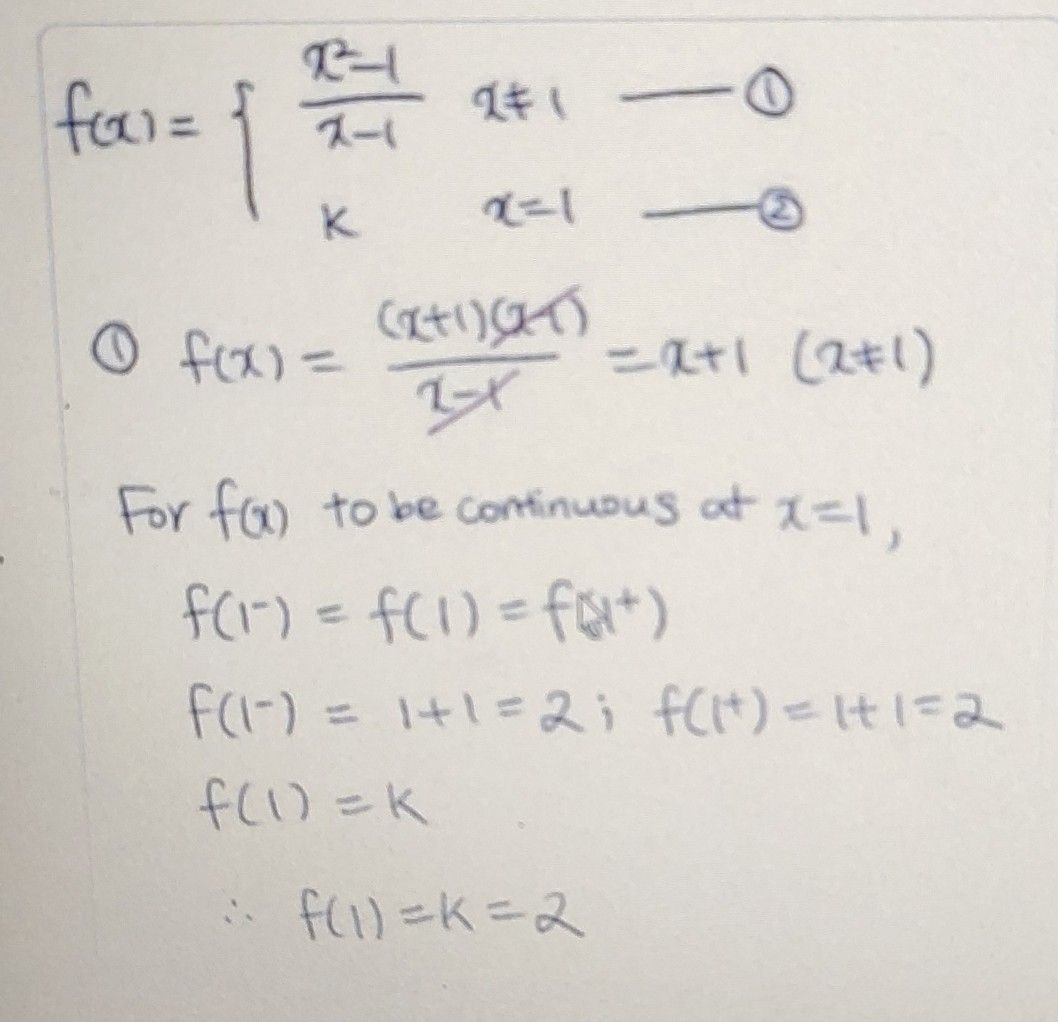Symbol
Problem$2.$ $3$ Evaluate $\int |x^{2}-2x|dx$ $1$ $3.$ $f\left(x\right)=\left(\dfrac {x^{2-1}} {x-1}$ $k$ when $x≠1$ If the function when $x=1$ is given to be continuous at $tx=1$ then the value of $k$ is
Physics
SolutionQanda teacher - UttamSingh